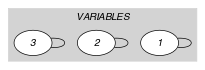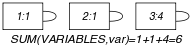## 5.384. sum_ctr

Origin

Arithmetic constraint.

Constraint

$\mathrm{𝚜𝚞𝚖}_\mathrm{𝚌𝚝𝚛}\left(\mathrm{𝚅𝙰𝚁𝙸𝙰𝙱𝙻𝙴𝚂},\mathrm{𝙲𝚃𝚁},\mathrm{𝚅𝙰𝚁}\right)$

Synonyms

$\mathrm{𝚌𝚘𝚗𝚜𝚝𝚊𝚗𝚝}_\mathrm{𝚜𝚞𝚖}$, $\mathrm{𝚜𝚞𝚖}$, $\mathrm{𝚕𝚒𝚗𝚎𝚊𝚛}$, $\mathrm{𝚜𝚌𝚊𝚕𝚊𝚛}_\mathrm{𝚙𝚛𝚘𝚍𝚞𝚌𝚝}$.

Arguments
 $\mathrm{𝚅𝙰𝚁𝙸𝙰𝙱𝙻𝙴𝚂}$ $\mathrm{𝚌𝚘𝚕𝚕𝚎𝚌𝚝𝚒𝚘𝚗}\left(\mathrm{𝚟𝚊𝚛}-\mathrm{𝚍𝚟𝚊𝚛}\right)$ $\mathrm{𝙲𝚃𝚁}$ $\mathrm{𝚊𝚝𝚘𝚖}$ $\mathrm{𝚅𝙰𝚁}$ $\mathrm{𝚍𝚟𝚊𝚛}$
Restrictions
 $\mathrm{𝚛𝚎𝚚𝚞𝚒𝚛𝚎𝚍}$$\left(\mathrm{𝚅𝙰𝚁𝙸𝙰𝙱𝙻𝙴𝚂},\mathrm{𝚟𝚊𝚛}\right)$ $\mathrm{𝙲𝚃𝚁}\in \left[=,\ne ,<,\ge ,>,\le \right]$
Purpose

Constraint the sum of a set of domain variables. More precisely, let $𝚂$ denote the sum of the variables of the $\mathrm{𝚅𝙰𝚁𝙸𝙰𝙱𝙻𝙴𝚂}$ collection (when the collection is empty the corresponding sum is equal to 0). Enforce the following constraint to hold: $𝚂\mathrm{𝙲𝚃𝚁}\mathrm{𝚅𝙰𝚁}$.

Example
$\left(〈1,1,4〉,=,6\right)$

The $\mathrm{𝚜𝚞𝚖}_\mathrm{𝚌𝚝𝚛}$ constraint holds since the condition $1+1+4=6$ is satisfied.

Typical
 $|\mathrm{𝚅𝙰𝚁𝙸𝙰𝙱𝙻𝙴𝚂}|>1$ $\mathrm{𝚛𝚊𝚗𝚐𝚎}$$\left(\mathrm{𝚅𝙰𝚁𝙸𝙰𝙱𝙻𝙴𝚂}.\mathrm{𝚟𝚊𝚛}\right)>1$ $\mathrm{𝙲𝚃𝚁}\in \left[=,<,\ge ,>,\le \right]$
Symmetry

Items of $\mathrm{𝚅𝙰𝚁𝙸𝙰𝙱𝙻𝙴𝚂}$ are permutable.

Arg. properties
• Contractible wrt. $\mathrm{𝚅𝙰𝚁𝙸𝙰𝙱𝙻𝙴𝚂}$ when $\mathrm{𝙲𝚃𝚁}\in \left[<,\le \right]$ and $\mathrm{𝚖𝚒𝚗𝚟𝚊𝚕}\left(\mathrm{𝚅𝙰𝚁𝙸𝙰𝙱𝙻𝙴𝚂}.\mathrm{𝚟𝚊𝚛}\right)\ge 0$.

• Contractible wrt. $\mathrm{𝚅𝙰𝚁𝙸𝙰𝙱𝙻𝙴𝚂}$ when $\mathrm{𝙲𝚃𝚁}\in \left[\ge ,>\right]$ and $\mathrm{𝚖𝚊𝚡𝚟𝚊𝚕}\left(\mathrm{𝚅𝙰𝚁𝙸𝙰𝙱𝙻𝙴𝚂}.\mathrm{𝚟𝚊𝚛}\right)\le 0$.

• Extensible wrt. $\mathrm{𝚅𝙰𝚁𝙸𝙰𝙱𝙻𝙴𝚂}$ when $\mathrm{𝙲𝚃𝚁}\in \left[\ge ,>\right]$ and $\mathrm{𝚖𝚒𝚗𝚟𝚊𝚕}\left(\mathrm{𝚅𝙰𝚁𝙸𝙰𝙱𝙻𝙴𝚂}.\mathrm{𝚟𝚊𝚛}\right)\ge 0$.

• Extensible wrt. $\mathrm{𝚅𝙰𝚁𝙸𝙰𝙱𝙻𝙴𝚂}$ when $\mathrm{𝙲𝚃𝚁}\in \left[<,\le \right]$ and $\mathrm{𝚖𝚊𝚡𝚟𝚊𝚕}\left(\mathrm{𝚅𝙰𝚁𝙸𝙰𝙱𝙻𝙴𝚂}.\mathrm{𝚟𝚊𝚛}\right)\le 0$.

• Aggregate: $\mathrm{𝚅𝙰𝚁𝙸𝙰𝙱𝙻𝙴𝚂}\left(\mathrm{𝚞𝚗𝚒𝚘𝚗}\right)$, $\mathrm{𝙲𝚃𝚁}\left(\mathrm{𝚒𝚍}\right)$, $\mathrm{𝚅𝙰𝚁}\left(+\right)$.

Remark

When $\mathrm{𝙲𝚃𝚁}$ corresponds to $=$ this constraint is referenced under the names $\mathrm{𝚌𝚘𝚗𝚜𝚝𝚊𝚗𝚝}_\mathrm{𝚜𝚞𝚖}$ in KOALOG (http://www.koalog.com/php/index.php) and $\mathrm{𝚜𝚞𝚖}$ in JaCoP (http://www.jacop.eu/).

Systems
Used in

assignment dimension added: $\mathrm{𝚒𝚗𝚝𝚎𝚛𝚟𝚊𝚕}_\mathrm{𝚊𝚗𝚍}_\mathrm{𝚜𝚞𝚖}$ (assignment dimension corresponding to intervals is added).

generalisation: $\mathrm{𝚜𝚌𝚊𝚕𝚊𝚛}_\mathrm{𝚙𝚛𝚘𝚍𝚞𝚌𝚝}$ (arithmetic constraint where all coefficients are not necessarly equal to 1).

Keywords
Cond. implications

$•$ $\mathrm{𝚜𝚞𝚖}_\mathrm{𝚌𝚝𝚛}\left(\mathrm{𝚅𝙰𝚁𝙸𝙰𝙱𝙻𝙴𝚂},\mathrm{𝙲𝚃𝚁},\mathrm{𝚅𝙰𝚁}\right)$

with  $\mathrm{𝚅𝙰𝚁𝙸𝙰𝙱𝙻𝙴𝚂}.\mathrm{𝚟𝚊𝚛}\ge 0$

and   $\mathrm{𝚅𝙰𝚁𝙸𝙰𝙱𝙻𝙴𝚂}.\mathrm{𝚟𝚊𝚛}\le 1$

implies $\mathrm{𝚜𝚞𝚖}_\mathrm{𝚜𝚚𝚞𝚊𝚛𝚎𝚜}_\mathrm{𝚌𝚝𝚛}$$\left(\mathrm{𝚅𝙰𝚁𝙸𝙰𝙱𝙻𝙴𝚂},\mathrm{𝙲𝚃𝚁},\mathrm{𝚅𝙰𝚁}\right)$

when  $\mathrm{𝚅𝙰𝚁𝙸𝙰𝙱𝙻𝙴𝚂}.\mathrm{𝚟𝚊𝚛}\ge 0$

and   $\mathrm{𝚅𝙰𝚁𝙸𝙰𝙱𝙻𝙴𝚂}.\mathrm{𝚟𝚊𝚛}\le 1$.

$•$ $\mathrm{𝚜𝚞𝚖}_\mathrm{𝚌𝚝𝚛}\left(\mathrm{𝚅𝙰𝚁𝙸𝙰𝙱𝙻𝙴𝚂},\mathrm{𝙲𝚃𝚁},\mathrm{𝚅𝙰𝚁}\right)$

with  $\mathrm{𝚅𝙰𝚁𝙸𝙰𝙱𝙻𝙴𝚂}.\mathrm{𝚟𝚊𝚛}\ge -1$

and   $\mathrm{𝚅𝙰𝚁𝙸𝙰𝙱𝙻𝙴𝚂}.\mathrm{𝚟𝚊𝚛}\le 1$

implies $\mathrm{𝚜𝚞𝚖}_\mathrm{𝚌𝚞𝚋𝚎𝚜}_\mathrm{𝚌𝚝𝚛}$$\left(\mathrm{𝚅𝙰𝚁𝙸𝙰𝙱𝙻𝙴𝚂},\mathrm{𝙲𝚃𝚁},\mathrm{𝚅𝙰𝚁}\right)$

when  $\mathrm{𝚅𝙰𝚁𝙸𝙰𝙱𝙻𝙴𝚂}.\mathrm{𝚟𝚊𝚛}\ge -1$

and   $\mathrm{𝚅𝙰𝚁𝙸𝙰𝙱𝙻𝙴𝚂}.\mathrm{𝚟𝚊𝚛}\le 1$.

$•$ $\mathrm{𝚜𝚞𝚖}_\mathrm{𝚌𝚝𝚛}\left(\mathrm{𝚅𝙰𝚁𝙸𝙰𝙱𝙻𝙴𝚂},\mathrm{𝙲𝚃𝚁},\mathrm{𝚅𝙰𝚁}\right)$

with  $\mathrm{𝚅𝙰𝚁𝙸𝙰𝙱𝙻𝙴𝚂}.\mathrm{𝚟𝚊𝚛}\ge -1$

and   $\mathrm{𝚅𝙰𝚁𝙸𝙰𝙱𝙻𝙴𝚂}.\mathrm{𝚟𝚊𝚛}\le 1$

implies $\mathrm{𝚜𝚞𝚖}_\mathrm{𝚙𝚘𝚠𝚎𝚛𝚜}\mathtt{5}_\mathrm{𝚌𝚝𝚛}$$\left(\mathrm{𝚅𝙰𝚁𝙸𝙰𝙱𝙻𝙴𝚂},\mathrm{𝙲𝚃𝚁},\mathrm{𝚅𝙰𝚁}\right)$

when  $\mathrm{𝚅𝙰𝚁𝙸𝙰𝙱𝙻𝙴𝚂}.\mathrm{𝚟𝚊𝚛}\ge -1$

and   $\mathrm{𝚅𝙰𝚁𝙸𝙰𝙱𝙻𝙴𝚂}.\mathrm{𝚟𝚊𝚛}\le 1$.

$•$ $\mathrm{𝚜𝚞𝚖}_\mathrm{𝚌𝚝𝚛}\left(\mathrm{𝚅𝙰𝚁𝙸𝙰𝙱𝙻𝙴𝚂},\mathrm{𝙲𝚃𝚁},\mathrm{𝚅𝙰𝚁}\right)$

with  $\mathrm{𝙲𝚃𝚁}\in \left[=\right]$

and   $\mathrm{𝚒𝚗𝚌𝚛𝚎𝚊𝚜𝚒𝚗𝚐}$$\left(\mathrm{𝚅𝙰𝚁𝙸𝙰𝙱𝙻𝙴𝚂}\right)$

implies $\mathrm{𝚒𝚗𝚌𝚛𝚎𝚊𝚜𝚒𝚗𝚐}_\mathrm{𝚜𝚞𝚖}$$\left(\mathrm{𝚅𝙰𝚁𝙸𝙰𝙱𝙻𝙴𝚂},\mathrm{𝚅𝙰𝚁}\right)$.

Arc input(s)

$\mathrm{𝚅𝙰𝚁𝙸𝙰𝙱𝙻𝙴𝚂}$

Arc generator
$\mathrm{𝑆𝐸𝐿𝐹}$$↦\mathrm{𝚌𝚘𝚕𝚕𝚎𝚌𝚝𝚒𝚘𝚗}\left(\mathrm{𝚟𝚊𝚛𝚒𝚊𝚋𝚕𝚎𝚜}\right)$

Arc arity
Arc constraint(s)
$\mathrm{𝚃𝚁𝚄𝙴}$
Graph property(ies)
$\mathrm{𝐒𝐔𝐌}$$\left(\mathrm{𝚅𝙰𝚁𝙸𝙰𝙱𝙻𝙴𝚂},\mathrm{𝚟𝚊𝚛}\right)\mathrm{𝙲𝚃𝚁}\mathrm{𝚅𝙰𝚁}$

Graph model

Since we want to keep all the vertices of the initial graph we use the $\mathrm{𝑆𝐸𝐿𝐹}$ arc generator together with the $\mathrm{𝚃𝚁𝚄𝙴}$ arc constraint. This predefined arc constraint always holds.

Parts (A) and (B) of Figure 5.384.1 respectively show the initial and final graph associated with the Example slot. Since we use the $\mathrm{𝚃𝚁𝚄𝙴}$ arc constraint both graphs are identical.

##### Figure 5.384.1. Initial and final graph of the $\mathrm{𝚜𝚞𝚖}_\mathrm{𝚌𝚝𝚛}$ constraint(a) (b)